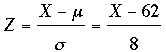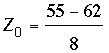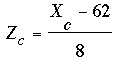SEARCH HOMEMath Central Quandaries & QueriesQuestion from EDGAR, a student: to qualify for security officers training recruits are tested for stress tolerance. The scores are normally distributed with mean of 62 and a standard deviation of 8. a.) If only the top 15% of recruits are selected, find the cutoff score b.) If a candidate is randomly selected, what is the probability that his or her socre is at least 55?Edgar,

First you need to convert the normal random variable X with mean μ = 62 and standard deviation σ = 8 to the standard normal random variable Z using(1)

For part b) findand use you standard normal table to find

Pr(Z ≥Zo)

For part a) find Zc so that

Pr(Z ≥ Zc) = 0.15

and then useto find the cut-off score Xc.

HarleyMath Central is supported by the University of Regina and The Pacific Institute for the Mathematical Sciences.